International
Tables for
Crystallography
Volume B
Reciprocal space
Edited by U. Shmueli

International Tables for Crystallography (2006). Vol. B. ch. 2.1, pp. 205-206   | 1 | 2 |

## Section 2.1.8.4. A more complicated example

U. Shmuelia* and A. J. C. Wilsonb

aSchool of Chemistry, Tel Aviv University, Tel Aviv 69 978, Israel, and bSt John's College, Cambridge, England
Correspondence e-mail:  ushmueli@post.tau.ac.il

#### 2.1.8.4. A more complicated example

| top | pdf |

We now illustrate the methodology of deriving characteristic functions for space groups of higher symmetries, following the method of Rabinovich et al. (1991a,b). The derivation is performed for the space group P[No. 174]. According to Table A1.4.3.6, the real and imaginary parts of the normalized structure factor are given by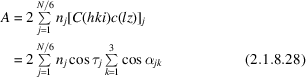and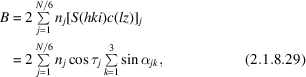where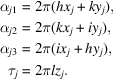Note that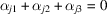, i.e., one of these contributions depends on the other two; this is a recurring problem in calculations pertaining to trigonal and hexagonal systems. For brevity, we write directly the general form of the characteristic function from which the functional form of the Fourier coefficient can be readily obtained. The characteristic function is given by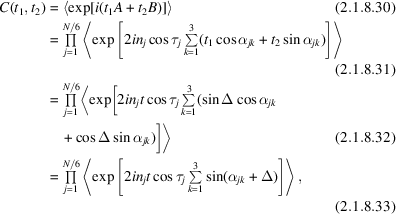where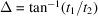,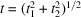and the assumption of independence was used. If we further employ the assumption of uniformity, while remembering that the angular variables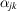are not independent, the characteristic function can be written as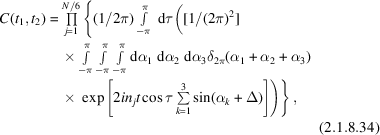where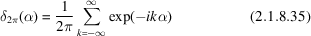is the Fourier representation of the periodic delta function. Equation (2.1.8.34)then becomes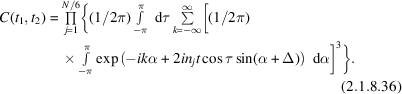If we change the variable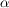to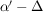,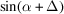becomes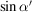and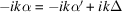. Hence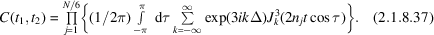The imaginary part of the summation, involving Bessel functions of odd orders, vanishes upon integration and the latter is restricted to the positive quadrant in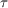. Thus, upon replacing cosines by sines (this is permissible at this stage) the atomic contribution to the characteristic function becomes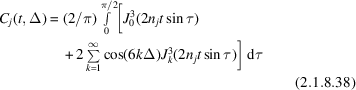and a double Fourier series must be used for the p.d.f.

### ReferencesRabinovich, S., Shmueli, U., Stein, Z., Shashua, R. & Weiss, G. H. (1991a). Exact random-walk models in crystallographic statistics. VI. P.d.f.'s offor all plane groups and most space groups. Acta Cryst. A47, 328–335.Google ScholarRabinovich, S., Shmueli, U., Stein, Z., Shashua, R. & Weiss, G. H. (1991b). Exact random-walk models in crystallographic statistics. VII. An all-space-group study of the effects of atomic heterogeneity on the p.d.f.'s of. Acta Cryst. A47, 336–340.Google Scholar